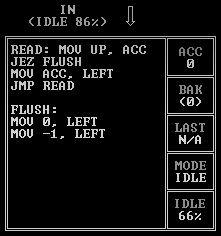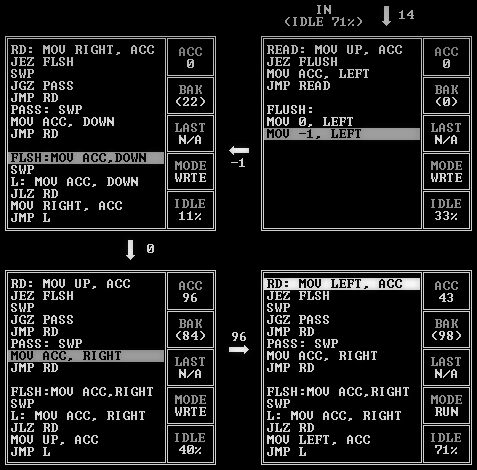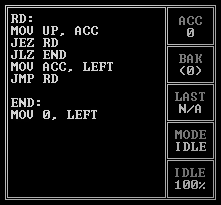## “TIS-100” – SEQUENCE REVERSER (SEGMENT 42656)

TIS-100 index

Solution for the most ridiculous achievement: NO_MEMORY, or reversing a list without using a stack memory node. This will probably be the longest post of the series.We need to read in the whole list to be able to reverse it, so where are we going to keep the numbers if we can’t use a memory node? Well, I guess the only option is to store them in the execution units!

The overall flow of the program looks like this:1. Data is passed along the snaking path from input to output, always forward.
2. The elements of the list are passed along the line and stored in the first unoccupied numbered unit they reach. So if the list is [22, 84, 98, 43, 96], then 22 will be stored in unit 1, 84 in unit 2, and so on. (There are five of these units because the maximum length of a list in the test data is five; there’s room for one more in the layout if it was necessary.)
3. Once we’ve done this, we’ve already reversed the list: the last element in the list is the one closest to the output, and the first element in the list is the one farthest away!
4. Now all we have to do is output the reversed list like a train: every number moves forward one unit until they reach the end.The input unit’s job is simple: when it reads in a list value, it sends it along, and when it reaches the end of the list, it sends out two special signal values, 0 followed by -1. More on this later.The code for each of the five numbered storage units is exactly same, except for the directions they read and write data from. They all start with a value of 0 in `BAK` at the start of every list cycle. There are basically two different states they go through: read/pass (step #2 in the list above) and flush (step #4).

In the read/pass state, they read in a value and check it. If the value is positive, that means it’s a number the unit has to store, so the first thing the unit does is `SWP` in the value from `BAK` and see if it was already storing a number. If `BAK` was 0 and it wasn’t, then it just saved a number to `BAK`, so everything’s done and we can restart the read/pass cycle. Otherwise, it `SWP`s the number it just read back out and passes it along to the next unit.

So as the flushing begins, we can see that unit 1 has the first value of the list, 22, stored in `BAK`, unit 2 has 84, and unit 3 has 98:If the unit reads in a value of 0 during the read/pass state, that’s the signal to start flushing (see earlier, when the input unit passes a 0 and a -1). The first thing a unit does when entering the flush state is pass that 0 signal along to the next unit; the 0 is fed along until it reaches the output unit, which throws it away. More on this later.

After that, the unit then `SWP`s out its stored value in order to pass it along. This instruction has a second vital purpose: since the flush signal of 0 was still in `ACC`, that 0 gets swapped into `BAK`, initializing the unit for the next read/pass cycle.

At this point, the unit enters a loop of passing its `ACC` value to the next unit and then reading a value from the previous unit into `ACC`. This continues until it passes out a negative number, after which the unit resets itself to the start of the read/pass cycle. Remember how the input unit’s flush signal is a 0 followed by a -1? That -1 is the last car of the train, used by every unit to know when the list is over:Here we can see the list queueing up, with -1 at the rear.

The output unit will first receive the number 0 (the flush signal), followed by all the values of the list in reverse order, followed by the -1 at the very end. So the code for this is very easy:If the value is positive, it sends it out. If the value is 0, it throws it away. And if the value is negative, it outputs a 0, because that means we’re at the end of the list. And that’s it!

Now, there is one more tricky bit here: there are five storage units, so what happens when the list we’re reversing has less than five numbers? Let’s see what happens when the list is only one number, .

In that case, when the flush signal of 0 is sent out, unit #1 be storing 38, but the value of `BAK` in the other four will all be the initial value, 0:The empty units that are holding on to 0s will behave in exactly the same way as units with values: they’ll swap in the contents from `BAK` and pass that forward. So in this case, the train will start with a bunch of 0s:Now, remember how the output unit throws away values of 0 it receives? That applies to these leading 0s too! So it’ll strip away all that crud until it reaches the first value of the list: 38. Which is exactly what we want.

That wasn’t so hard, was it?!